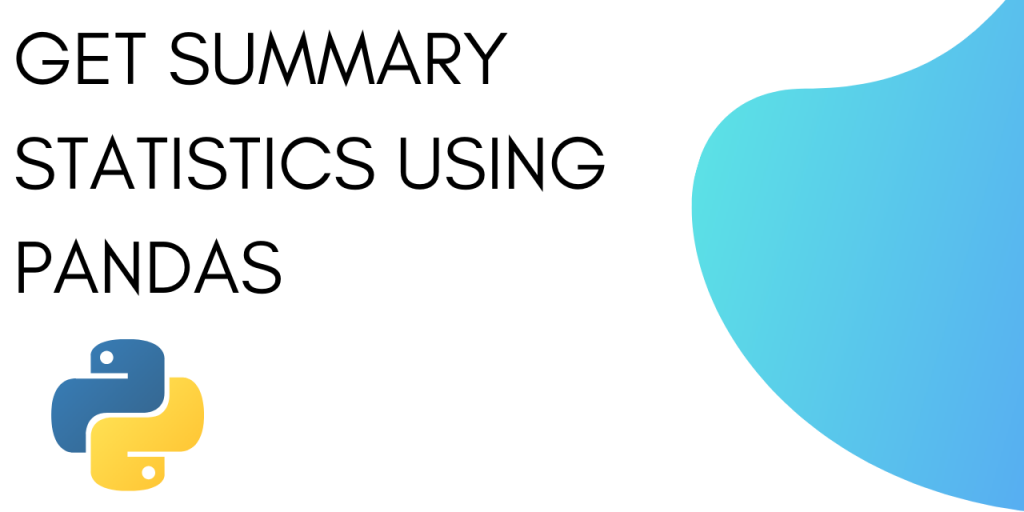# How to Calculate Summary Statistics in Python?To calculate summary statistics in Python you need to use the .describe() method under Pandas. The .describe() method works on both numeric data as well as object data such as strings or timestamps.

The output for the two will contain different fields. For numeric data the result will include:

For object data the result will include :

• count
• unique
• top
• freq

## Calculate Summary Statistics in Python Using the describe() method

In this tutorial, we will see how to use .describe() method with numeric and object data.

We will also see how to analyze a large dataset and timestamp series using .describe method.

Let’s get started.

### 1. Summary Statistics for Numeric data

Let’s define a list with numbers from 1 to 6 and try getting summary statistics for the list.

We will start by importing pandas.

```import pandas as pd
```

Now we can define a series as :

```s = pd.Series([1, 2, 3, 4, 5, 6])
```

To display summary statistics use:

```s.describe()
```

The complete code and output are as follows :

```import pandas as pd
s = pd.Series([1, 2, 3, 4, 5, 6])
s.describe()
```

Output :

```count    6.000000
mean     3.500000
std      1.870829
min      1.000000
25%      2.250000
50%      3.500000
75%      4.750000
max      6.000000
dtype: float64
```

Let’s understand what each of the value means.

### 2. Summary Statistics for Python Object data

Let’s define a series as a set of characters and use the .describe method on it to calculate summary statistics.

We can define the series as:

```s = pd.Series(['a', 'a', 'b', 'c'])
```

To get the summary statistics use :

```s.describe()
```

The complete code and output is as follows:

```import pandas
s = pd.Series(['a', 'a', 'b', 'c'])
s.describe()
```

Output:

```count     4
unique    3
top       a
freq      2
dtype: object
```

Let’s understand what each of the following means:

### 3. Summary statistics of a large data set

You can use pandas to get the summary statistics from a large dataset as well. You just need to import the dataset into a pandas data frame and then use the .describe method.

In this tutorial, we will be using the California Housing dataset as the sample dataset.

Let’s start by importing the CSV dataset and then call the .describe method on it.

```import pandas as pd
housing.describe()
```

Output :

We can see that the result contains the summary statistics for all the columns in our dataset.

### 4. Summary Statistics for timestamp series

You can use .describe to get summary statistics for a timestamp series as well. Let’s start by defining a timestamp series.

```import datetime
import numpy as np
s = pd.Series([np.datetime64("2000-01-01"),np.datetime64("2010-01-01"),np.datetime64("2010-01-01"),np.datetime64("2002-05-08")])
```

Now you can call .describe on this timestamp series.

``` s.describe()
```

The complete code and output are as follows :

```import datetime
import numpy as np
s = pd.Series([np.datetime64("2000-01-01"),np.datetime64("2010-01-01"),np.datetime64("2010-01-01"),np.datetime64("2002-05-08")])
s.describe()
```

Output:

```count                       4
unique                      3
top       2010-01-01 00:00:00
freq                        2
first     2000-01-01 00:00:00
last      2010-01-01 00:00:00
dtype: object
```

You can also instruct .describe to treat dateTime as a numeric. This will display the result in a manner similar to that of numeric data. You can get mean, median, 25 percentile and 75 percentile in DateTime format.

This can be done using :

```s.describe(datetime_is_numeric=True)
```

The output is as follows:

```count                      4
mean     2005-08-03 00:00:00
min      2000-01-01 00:00:00
25%      2001-10-05 12:00:00
50%      2006-03-05 12:00:00
75%      2010-01-01 00:00:00
max      2010-01-01 00:00:00
```

You can see that the result contains mean, median, 25 percentile and 75 percentile in DateTime format.

## Conclusion

This tutorial was about computing summary statistics in Python. We looked at numeric data, object data, large datasets and timestamp series to calculate summary statistics.